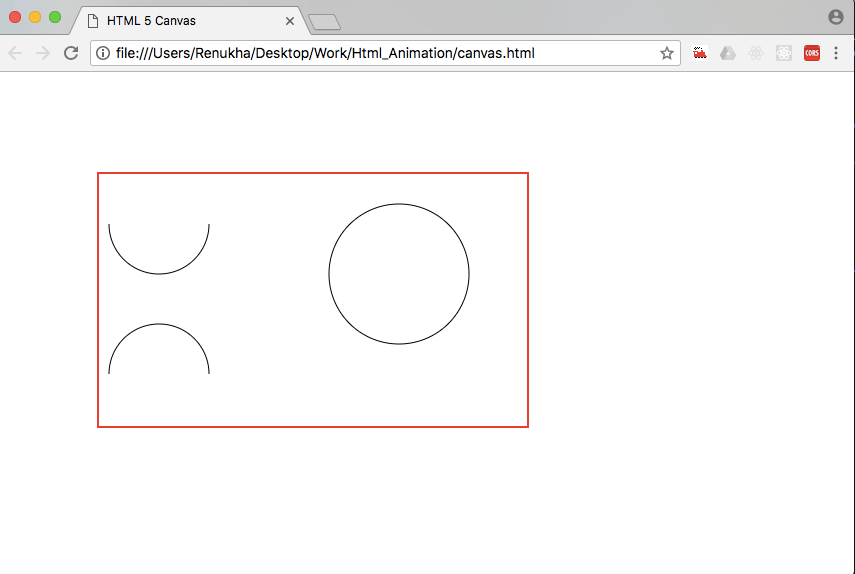# How to draw a circle in Canvas?

We can draw a circle in Canvas using the  arc(x,y,r,startAngle,endAngle,counterClockwise)  method. The arc method is used to create curves or circles using the startAngle and endAngle values.The beginPath()  method begins the path or resets the current path. The arc method draws a circle with a center point specified by x, y and radius r. The start angle denotes the angle from which we have to start drawing the arc and the end angle denotes the angle till which we continue drawing the arc. For a circle, the startAngle can be at 0° and endAngle at 360°. In terms of radians, startAngle is given by 0*Math.PI and endAngle is given by 2*Math.PI. The stroke() method is used to actually draw the path on the canvas. The counterClockwise denotes whether the path should be drawn  in clockwise or anticlockwise direction.By default, it is set to false indicating that the path must be drawn in clockwise direction.